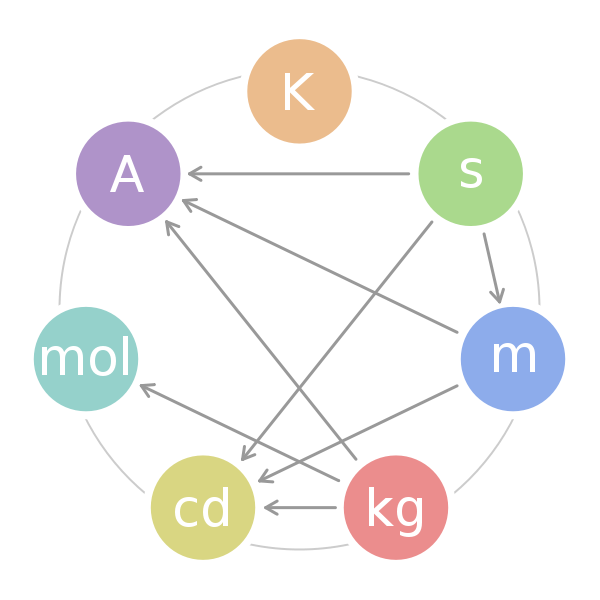## Standard Units (SI Units)

#### Learning Objective

• Recognize SI units and their importance for measurment

#### Key Points

• Every field of science involves taking measurements, understanding them, and communicating them to others. In other words, we all have to speak the same basic language.
• The SI system, also called the metric system, is used around the world.
• There are seven basic units in the SI system: the meter (m), the kilogram (kg), the second (s), the kelvin (K), the ampere (A), the mole (mol), and the candela (cd).

#### Term

• SI systemA series of units that is accepted and used throughout the scientific world.

## The Need for a Common Language

Every field of science involves taking measurements, understanding them, and communicating them to others. In other words, we all have to speak the same basic language. Whether you are a chemist, a physicist, a biologist, an engineer, or even a medical doctor, you need a consistent way of communicating size, mass, shape, temperature, time, amount, energy, power, and speed.

Consider the screen on which you’re reading this text right now. It might be an LCD screen, which is made up of liquid crystals. The chemist developing a specific formulation for a liquid crystal has to meaningfully communicate information to an engineer so that the engineer knows how to manufacture it. The engineer, in turn has to be able to communicate with other engineers, physicists, and chemists to design the circuit boards, display screens, and electronic interfaces of the rest of the computer. If these people don’t all speak the same language, the enterprise will never get off the ground.

The International System of Units (abbreviated SI, from the French Système international d’unités) is the metric system used in science, industry, and medicine. Depending on your age and geographic location, you might be very familiar with the “imperial” system, which includes units such as gallons, feet, miles, and pounds. The imperial system is used for “everyday” measurements in a few places, such as the United States. But in most of the world (including Europe) and in all scientific circles, the SI system is in common use.

## Units of the SI System

There are seven base units in the SI system:

• the kilogram (kg), for mass
• the second (s), for time
• the kelvin (K), for temperature
• the ampere (A), for electric current
• the mole (mol), for the amount of a substance
• the candela (cd), for luminous intensity
• the meter (m), for distanceThe Seven SI UnitsThis figure displays the fundamental SI units and the combinations that lead to more complex units of measurement.

It should be apparent that the move into modern times has greatly refined the conditions of measurement for each basic unit in the SI system, making the measurement of, for example, the luminous intensity of a light source a standard measurement in every laboratory in the world. A light source made to produce 20 cd will be the same regardless of whether it is made in the United States, in the UK, or anywhere else. The use of the SI system provides all scientists and engineers with a common language of measurement.

## History of the SI System

The SI units of measurement have an interesting history. Over time they have been refined for clarity and simplicity.

• The meter (m), or metre, was originally defined as 1/10,000,000 of the distance from the Earth’s equator to the North Pole measured on the circumference through Paris. In modern terms, it is defined as the distance traveled by light in a vacuum over a time interval of 1/299,792,458 of a second.
• The kilogram (kg) was originally defined as the mass of a liter (i.e., of one thousandth of a cubic meter). It is currently defined as the mass of a platinum-iridium kilogram sample maintained by the Bureau International des Poids et Mesures in Sevres, France.
• The second (s) was originally based on a “standard day” of 24 hours, with each hour divided in 60 minutes and each minute divided in 60 seconds. However, we now know that a complete rotation of the Earth actually takes 23 hours, 56 minutes, and 4.1 seconds. Therefore, a second is now defined as the duration of 9,192,631,770 periods of the radiation corresponding to the transition between the two hyperfine levels of the ground state of the cesium-133 atom.
• The ampere (A) is a measure of the amount of electric charge passing a point in an electric circuit per unit time. 6.241×1018electrons, or one coulomb, per second constitutes one ampere.
• The kelvin (K) is the unit of the thermodynamic temperature scale. This scale starts at 0 K. The incremental size of the kelvin is the same as that of the degree on the Celsius (also called centigrade) scale. The kelvin is the fraction 1/273.16 of the thermodynamic temperature of the triple point of water (exactly 0.01 °C, or 32.018 °F).
• The mole (mol) is a number that relates molecular or atomic mass to a constant number of particles. It is defined as the amount of a substance that contains as many elementary entities as there are atoms in 0.012 kg of carbon-12.
• The candela (cd) was so named to refer to “candlepower” back in the days when candles were the most common source of illumination (because so many people used candles, their properties were standardized). Now, with the prevalence of incandescent and fluorescent light sources, the candela is defined as the luminous intensity in a given direction of a source that emits monochromatic radiation of frequency $540 \cdot 10^{12}$ Hertz and that has a radiant intensity in that direction of 1/683 watts per steradian.